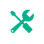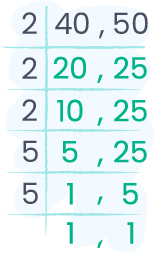# LCM CALCULATOR

To use this Least Common Multiple Calculator (LCM Calculator), Enter value in the first input (comma separated), select method and click on calculate button.
How it Works?
1. 1. Enter numbers in first input box separated by comma
2. 2. Select Calculation Method from dropdown
3. 3. Click on calculate button
LCM of
302
Finding LCM by Using Prime Factorization Method
Step 11
300 = 2 x 2 x 3 x 5 x 5
300 = 2 x 2
300 = 2 x 2 x 3
Step 2
300 = 22 x 3 x 52
30 = 2 x 3 x 5
Step 3
= 22 x 52 x 3
= 4 x 25 x 3
LCM(300, 30) = 300
Finding LCM by Using Division Table Method
Step 11
 2 30 300 2 15 150 3 15 75 5 5 25 5 1 5 1 1
Step 2
2 * 2 * 3 * 5 * 5
Step 3
LCM = 300
Finding LCM by List of Multiplies Method
Multiples of 300: 300 600 900
Multiples of 300: 300 600 900 600 900 600 900 600 900 600 900 600 900
LCM(300,30) = 300

## LCM Calculator

The online LCM calculator can find the least common multiple (factors) quickly than manual methods. Whether finding the LCM of two numbers or multiple numbers, this calculator can help you with just a single click.Related Tool Name

## What is the Least common factor (LCM)?

The least common multiple or lowest common multiples is the least number which is divisible by the numbers for which you are finding the LCM.

For example:
The L.C.M of 3, 5 and 6 is 30.
This means that the 30 is the common number that can be divided by any of the numbers between 3,5 and 6 to get the answer in integers.

## Methods of finding the LCM

There are multiple methods of finding the Lowest common multiple between multiple numbers. Some of the ways are discussed below:

• Listing Multiples
• Prime Factorization
• Prime Factorization using exponents
• Cake Method (also known as ladder method)
• Division Method

## How to find the LCM manually?

As discussed above, there are several methods to find the LCM. Let’s go through the important ones to understand the basic idea.

### 1. Prime Factorization

Prime factorization is a simple method to find the LCM. Let’s see how to find LCM using prime factorization step by step.

#### Example:

Find LCM of 400, 500, and 550 with prime factorization?
Solution:
Step 1: List the factors of the given numbers.
400: 5 × 5 × 2 × 2 × 2 × 2 = 52 × 24
500: 5 × 5 × 5 × 2 × 2 = 53 × 2 2
550: 5 × 5 × 2 × 11 = 52 × 2 × 11
Step 2: Multiply the prime factors to get the LCM. If there are common factors, use them only once.
53 × 24 × 11 = 22000
So, the least common multiple of 400, 500, and 550 is 22000.

### 2. Division Method

The division method is another method to get the LCM of multiple numbers. Follow the below steps to obtain the least common multiple using the division method.

#### Example:

Find the least common multiple of 40 and 50 with the division method?
Step 1: Place all numbers on a horizontal line as shown in the image below.Step 2: Start dividing the numbers with the least prime number. Write the quotient of division exactly below the dividend. Keep dividing until all numbers are fully divided and the remainder is 1.Step 3: Multiply all of the divisors to get the LCM.
2 × 2 × 2 × 5 × 5 = 200
So, the LCM of 40 and 50 is 200 using the division method.

### 3. Listing Multiples

The simplest way to find the LCM of any numbers is to find through the listing multiples. In this method, you have to list down all the multiple of the numbers and then find the common multiple between these numbers which would be the LCM.

#### Example:

Find the LCM of 8 and 14 using lists of multiple method?
Step 1: Write down the multiples of the given numbers.
Multiples of 8 = 8, 16, 24, 32, 40, 48, 56, 64, 72
Multiples of 14 = 14, 28, 42, 56, 70, 84
Step 2: Look out for the common multiple in the multiples of all numbers.
Multiples of 8 = 8, 16, 24, 32, 40, 48, 56, 64, 72
Multiples of 14 = 14, 28, 42, 56, 70, 84
As we can see that the common number between the multiples of 8 and 14 is 56, which is the least common factor for these numbers.
LCM of 8 and 14 = 56

## Properties of LCM

• It is associative
• It is commutative
• It is distributive

## LCM Formula

#### Finding the LCM through GCF

Finding the least common multiple with the value of the greatest common factor is possible with the following formula:
`LCM(a,b) = (a x b) / GCF(a,b)`
Example:
The LCM of (8,14)
GCF(8,14) = 2
Using formula: (8 x 14) / 2
LCM = 56

## LCM Examples

lcm of 9 and 12 36 Convert
lcm of 8 and 14 56 Convert
lcm of 9 and 15 45 Convert
lcm of 8 and 12 24 Convert
lcm of 6 and 9 18 Convert
lcm of 12 and 15 60 Convert
lcm of 8 and 10 40 Convert
lcm of 4 and 6 12 Convert
lcm of 10 and 12 60 Convert
lcm of 6 and 12 12 Convert
lcm of 15 and 20 60 Convert
lcm of 12 and 18 36 Convert
lcm of 16 and 20 80 Convert
lcm of 24 and 30 120 Convert
lcm of 8,12 and 16 48 Convert
lcm of 9,10 and 28 1260 Convert